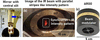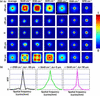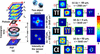## short communicationsJOURNAL OFSYNCHROTRONRADIATION
ISSN: 1600-5775
Volume 28| Part 5| September 2021| Pages 1616-1619

## Exploiting spatio-spectral aberrations for rapid synchrotron infrared imaging

aOptical Sciences Center, Swinburne University of Technology, John Street, Melbourne, Victoria 3122, Australia, bInfrared Microspectroscopy (IRM) Beamline, ANSTO – Australian Synchrotron, Clayton, Victoria 3168, Australia, and cTokyo Tech World Research Hub Initiative, School of Materials and Chemical Technology, Tokyo Institute of Technology, 2-12-1, Ookayama, Meguro-ku, Tokyo 152-8550, Japan
*Correspondence e-mail: vanand@swin.edu.au, jitrapov@ansto.gov.au, sjuodkazis@swin.edu.au

(Received 17 February 2021; accepted 9 July 2021; online 9 August 2021)

The Infrared Microspectroscopy Beamline at the Australian Synchrotron is equipped with a Fourier transform infrared (FTIR) spectrometer, which is coupled with an infrared (IR) microscope and a choice of two detectors: a single-point narrow-band mercury cadmium telluride (MCT) detector and a 64 × 64 multi-pixel focal plane array (FPA) imaging detector. A scanning-based point-by-point mapping method is commonly used with a tightly focused synchrotron IR beam at the sample plane, using an MCT detector and a matching 36× IR reflecting objective and condenser (NA = 0.5), which is time consuming. In this study, the beam size at the sample plane was increased using a 15× objective and the spatio-spectral aberrations were investigated. A correlation-based semi-synthetic computational optical approach was applied to assess the possibilities of exploiting the aberrations to perform rapid imaging rather than a mapping approach.

### 1. Introduction

The Infrared Microspectroscopy (IRM) Beamline at the Australian Synchrotron has a Fourier transform infrared (FTIR) micro-spectroscopic system that is equipped with a 64 × 64 multi-pixel focal plane array (FPA) imaging detector (Tobin et al., 2018). The IR beam is extracted from the storage ring by a mirror with a central slit as shown in Fig. 1(a) and continues as a beam resembling two parallel stripes as shown in Fig. 1(b). The FTIR microscope consists of annular reflective objective optics (AROO) to avoid chromatic aberrations prevalent in optical systems with refractive and diffractive lenses. The mounts of the AROO consist of cross-shaped supports as shown in Fig. 1(c) which in addition to the parallel stripes beam produces interesting focal characteristics.Figure 1 (a) Gold-coated plane mirror with a central slit. (b) Synchrotron-IR beam with an intensity pattern resembling parallel stripes. (c) 15× AROO.

In the standard transmission measurement as previously reported (Ryu et al., 2017), a matching 36× Schwarzschild IR reflecting objective and condenser (NA = 0.5) are used to focus the beam and a scanning approach involving a point-by-point mapping was applied to record a hyperspectral chemical image of the sample. This approach is time consuming and limits the beam time availability for many samples. In this study, the 36× IR reflecting objective and condenser were replaced with a set of matching 15× objective and condenser (NA = 0.4) to increase the area of illumination on the sample plane, with a sampling area of 2.8 µm × 2.8 µm per pixel. Due to the nature of high brilliance and high collimation of the synchrotron-IR beam, the readout active area on the FPA imaging detector was cropped from the full frame of 64 × 64 pixels to 32 × 32 pixels. Under this optical configuration, the size of the beam at the sample plane was ∼60 µm × 60 µm. The original output from the operating software (OPUS 8.0, Bruker Optik GmbH, Ettlingen, Germany) was converted into data point table (.dpt) files and loaded into MATLAB (R2019a, Mathworks, Natick, MA, USA), processed and converted into images. Six spectral channels (νn), namely 3640 cm−1 (n = 100), 3130 cm−1 (n = 300), 2610 cm−1 (n = 500), 2100 cm−1 (n = 700), 1580 cm−1 (n = 900) and 1070 cm−1 (n = 1100), were selected and about 200 images corresponding to n − 100 to n + 99 channels were averaged to obtain the image for the six channels. The variable n indicates the spectral channel number.

### 2. Spatio-spectral imaging characteristics

The imaging characteristics of the system were investigated by introducing a point object with a diameter of 5 µm. The averaged point spread function (PSF) for the above spectral channels and for an axial aberration Δz = ±50 µm in steps of 25 µm are shown in Fig. 2(a), assuming symmetry in the four quadrants of the image. The modulation transfer function (MTF) was calculated as. The plots of the MTF for three different spectral channels at Δz = −50 µm, 0 µm, 50 µm are shown in Fig. 2(b), indicating that the lower wavenumbers and higher aberrations suppress higher spatial frequencies, which led to a lower imaging resolution.Figure 2 (a) Images of the PSFs corresponding to different wavenumbers and axial aberrations, and (b) plots of MTFs of Δz = −50 µm, 2100 cm−1 (black), Δz = 0, 3640 cm−1 (green), Δz = 50 µm, 3640 cm−1 (pink).

### 3. Indirect imaging

The synchrotron IRM beamline is a spatially incoherent system where the light emitted by a point in the object plane does not interfere with light emitted from another point, but their intensities add up (Rosen et al., 2019). For simplicity, the entire imaging system is approximated [as shown in Fig. 3(a)] consisting of a complex amplitude modulator with u and v as the object and image distances. The intensity of PSF can be expressed as IPSF = |[CQ(1/u)Mexp(iΦ)]⊗Q(1/ν)|2, where C is the complex constant, Q(a) is the quadratic phase function given by, M is the amplitude mask of cross-shaped support, Φ is the phase introduced by the annular lens and ⊗ is 2D convolutional operator. An object consisting of m points can be expressed as a collection of delta functions. The imaging system maps every object point into intensity patterns as shown in Fig. 2(a). For an object consisting of two points, the resulting intensity pattern is a superposition as shown in Fig. 3(b). The recorded image is given as IO = IPSFo. The image of the object can be reconstructed as IR = IO * IPSF = oIPSF * IPSF, where  *' is a 2D correlation operator andis the auto-correlation function, which samples the object function. Recent studies on cross-correlation revealed that a non-linear correlation filter yielded a better signal-to-noise ratio (SNR) on the acquired spectra. The non-linear correlation is given aswhere a and b are tuned between −1 and 1, to obtain the minimum entropy given aswhere; (m,n) are the indexes of the correlation matrix and C(m,n) is the correlation distribution.Figure 3 (a) Optical configuration. (b) Image formation of two object points observed for two conditions: Δz = 50 µm, 3640 cm−1 and Δz = 0 µm, 1070 cm−1. Generation of aberrated images of π' and their reconstruction for (c) Δz = −50 µm, 2100 cm−1 and (d) Δz = 0 µm, 3640 cm−1. Generation of aberrated image of α' and its reconstruction for (e) Δz = 50 µm, 3640 cm−1. The green, blue, yellow and pink borders indicate PSF, object, aberrated image of object and reconstruction, respectively. The symbols ⊗' and  *' are 2D convolution and 2D non-linear correlation for (a = 0, b = 0.54) operators.

The proposed system may be considered a hybrid imaging system consisting of both direct as well as indirect imaging modes. For cases where a point in the object plane is mapped to a focused point in the image plane, the system acts as a direct imager. When a point in the object plane is mapped to a complicated intensity pattern, an indirect imaging method based on cross-correlation can be applied. For a thick object (≥50 µm) studied in this system, layer-by-layer 3D scanning is needed in the scanning mode of operation. In the proposed non-scanning approach, a single camera shot is sufficient. Two Greek letters namely π' and α' are used as virtual test objects. The formation of the aberrated image of π' for Δz = −50 µm, 2100 cm−1, Δz = 0 µm, 3640 cm−1 and the corresponding reconstructions (a = 0, b = 0.54) are shown in Figs. 3(c) and 3(d), respectively. The formation of an aberrated image of α' for Δz = 50 µm, 3640 cm−1 and the corresponding reconstruction (a = 0, b = 0.54) are shown in Fig. 3(e).

### 4. Conclusions

The spatio-spectral characteristics of the synchrotron-IR system were studied. A semi-synthetic analysis of the system revealed the possibilities of non-scanning multi-plane imaging using an FPA detector. The mathematical approaches proposed in this study demonstrate a potential for further development of a synchrotron-IR holography system for rapid FPA-aided 3D imaging of thick samples in a non-scanning mode, thereby substantially reducing the measurement time and enhancing productivity in terms of number of samples being analyzed within a limited beam time.

The MATLAB code for reading .dpt' file output from the OPUS software, converting the file into a spatio-spectral matrix library cataloging for different spectral channels, averaging and reconstructing the image using non-linear correlation is given as a text file in the supporting information.

### Funding information

This research was undertaken on the IRM beamline at the Australian Synchrotron (Victoria, Australia), part of ANSTO (proposal No. 15775, reference No. AS1/IRM/15775). This work was performed in part at the Swinburne's Nanofabrication Facility (Nanolab).

### ReferencesJOURNAL OFSYNCHROTRONRADIATION